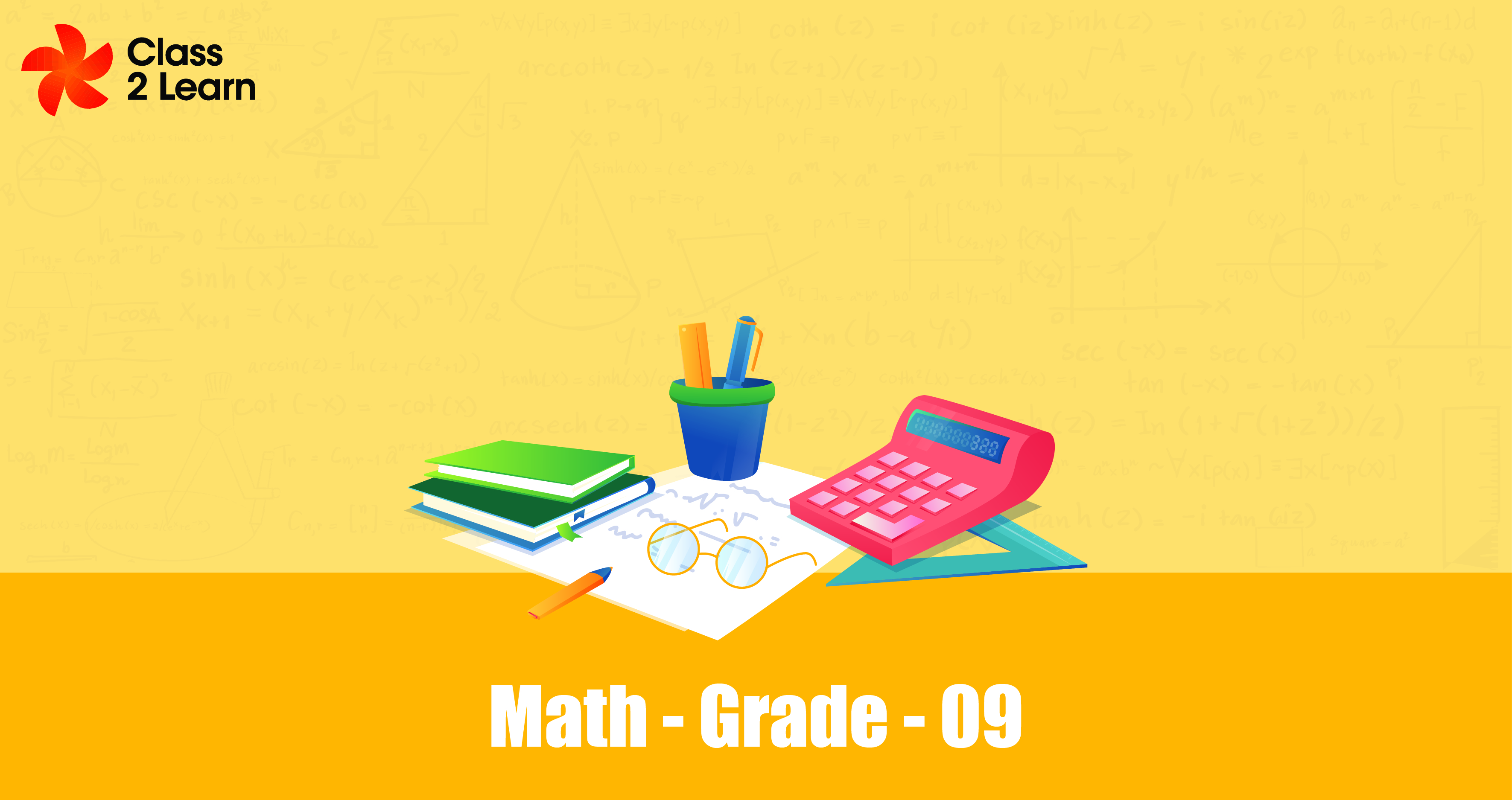# MathematicsMonthly One-time
Price
\$ 10

Per month

#### Overview

Mathematics is a systematic and abstract discipline that deals with the study of numbers, quantities, structures, and patterns. It encompasses various branches, including arithmetic, algebra, geometry, trigonometry, calculus, statistics, and more. At its core, mathematics involves logical reasoning and precise

#### Number System

The number system is a fundamental concept in mathematics that provides a way to represent and manipulate numbers. It defines different types of numbers and their properties, allowing us to perform calculations and solve mathematical problems.

#### Polynomials

Polynomials are fundamental mathematical expressions that involve variables raised to non-negative integer powers and combined using addition, subtraction, and multiplication operations. They are widely used in various branches of mathematics, science, engineering, and economics to model and analyze relationships between variables

#### Coordinate Geometry

Coordinate geometry, also known as analytic geometry, is a branch of mathematics that combines algebraic techniques with the principles of geometry. It provides a powerful framework for studying geometric figures using algebraic equations and numerical coordinates.

#### Linear Equation in Two Variables

Linear equations in two variables are algebraic equations that involve two unknowns or variables and can be represented graphically as straight lines on a coordinate plane. The main goal when working with linear equations with two variables is to find a solution that satisfies both equations simultaneously.

#### Lines and Angles

Lines and angles are fundamental concepts in geometry that describe the relationship between straight lines and the measures of angle formed by those lines. Understanding lines and angles is essential in various branches of mathematics and has practical applications in fields such as architecture and engineering.

A quadrilateral is a polygon with four sides. It is a fundamental shape in geometry, and understanding its properties and classifications is important in various mathematical and practical applications

#### Triangles

A triangle is a fundamental geometric figure that consists of three line segments connected to form a closed figure. It is the simplest polygon, as it has the fewest number of sides and vertices. Triangles are widely studied and have numerous applications in mathematics, physics, and other fields.

#### Circles

A circle is a geometric figure that is defined as a set of points in a plane that are equidistant from a fixed point called the center. It is one of the most studied and important shapes in mathematics.

#### Surface Area and Volume

The surface area and volume chapter in mathematics focuses on the calculation and understanding of the surface area and volume of various geometric figures, such as cubes, cylinders, spheres, cones, and prisms. It is an important topic in both geometry and real world applications, as it helps in solving problems related to the measurement and estimation of space occupied by objects.

#### Statistics

Statistics is a branch of mathematics that deals with the collection, analysis, interpretation, presentation, and organization of data. It involves the study of data patterns, variability, and relationships to make informed decisions and predictions.

#### Benefits

##### Included in this plan
• e-books
• Test Series & Analysis
• Assignments & Notes
• Hands on Activity
Free Trial Class

Take advantage of our free trial!